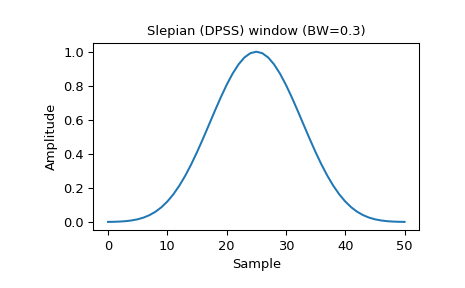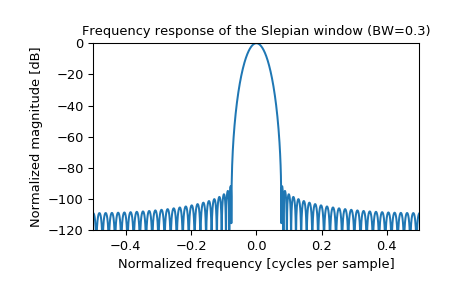# scipy.signal.windows.slepian¶

scipy.signal.windows.slepian(M, width, sym=True)[source]

Return a digital Slepian (DPSS) window.

Used to maximize the energy concentration in the main lobe. Also called the digital prolate spheroidal sequence (DPSS).

Note

Deprecated in SciPy 1.1. slepian will be removed in a future version of SciPy, it is replaced by dpss, which uses the standard definition of a digital Slepian window.

Parameters
Mint

Number of points in the output window. If zero or less, an empty array is returned.

widthfloat

Bandwidth

symbool, optional

When True (default), generates a symmetric window, for use in filter design. When False, generates a periodic window, for use in spectral analysis.

Returns
wndarray

The window, with the maximum value always normalized to 1

References

1

D. Slepian & H. O. Pollak: “Prolate spheroidal wave functions, Fourier analysis and uncertainty-I,” Bell Syst. Tech. J., vol.40, pp.43-63, 1961. https://archive.org/details/bstj40-1-43

2

H. J. Landau & H. O. Pollak: “Prolate spheroidal wave functions, Fourier analysis and uncertainty-II,” Bell Syst. Tech. J. , vol.40, pp.65-83, 1961. https://archive.org/details/bstj40-1-65

Examples

Plot the window and its frequency response:

>>> from scipy import signal
>>> from scipy.fft import fft, fftshift
>>> import matplotlib.pyplot as plt

>>> window = signal.slepian(51, width=0.3)
>>> plt.plot(window)
>>> plt.title("Slepian (DPSS) window (BW=0.3)")
>>> plt.ylabel("Amplitude")
>>> plt.xlabel("Sample")

>>> plt.figure()
>>> A = fft(window, 2048) / (len(window)/2.0)
>>> freq = np.linspace(-0.5, 0.5, len(A))
>>> response = 20 * np.log10(np.abs(fftshift(A / abs(A).max())))
>>> plt.plot(freq, response)
>>> plt.axis([-0.5, 0.5, -120, 0])
>>> plt.title("Frequency response of the Slepian window (BW=0.3)")
>>> plt.ylabel("Normalized magnitude [dB]")
>>> plt.xlabel("Normalized frequency [cycles per sample]")